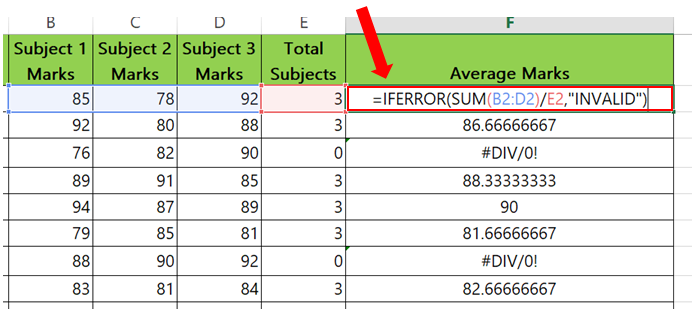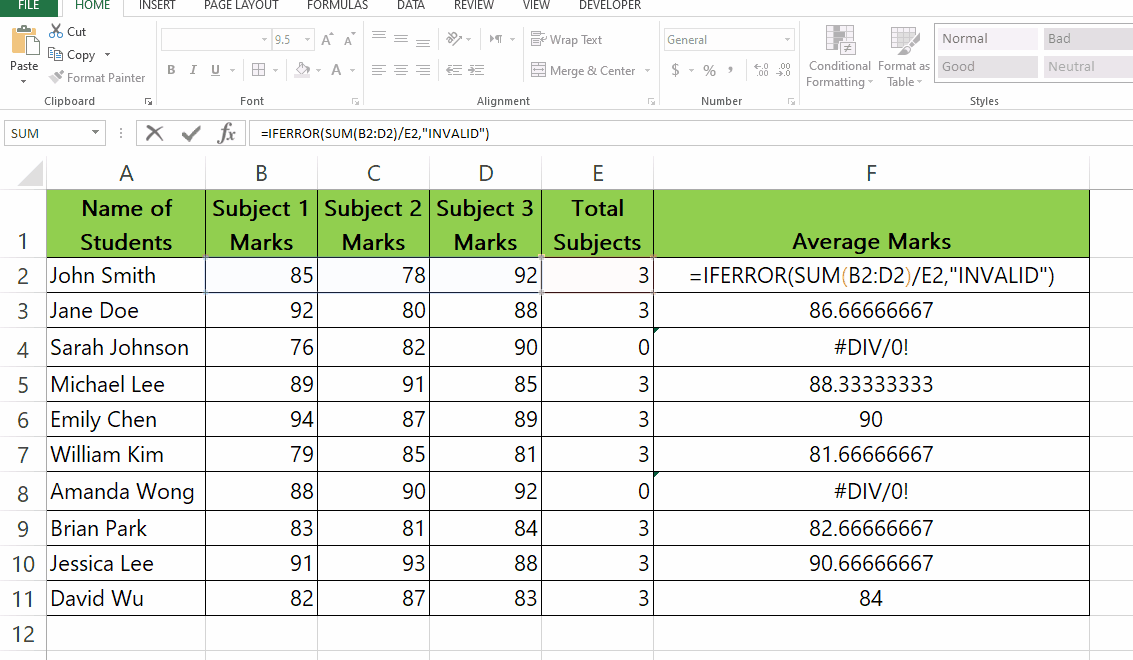# How to Remove DIV/0 Errors in Excel

In this this tutorial we learn how to remove this error from Excel by following the steps below but first let’s have a look at an example dataset with #DIV/0! Error. This dataset above is related to school which contains student Marks of Different Subjects, Total Subjects and Average Marks.When you perform calculations in Excel, you may sometimes encounter the “#DIV/0!” error message. This error occurs when a formula attempts to divide a number by zero, which is not a valid operation. While the error message itself can be useful in some cases, it can also be distracting and make it difficult to read or interpret your data.

### Step 1 – Use the formula given below.– To remove #DIV/0! Error from the Average Marks column we will use a different formula.
– Syntax of the formula is
=IFERROR(Formula, Value in place of #DIV/0! error )
– In our case the formula will be
=IFERROR( SUM(B2:D2)/E2, “INVALID” )
– This formula will change all #DIV/0! errors to INVALID.

### Step 2 – Apply the formula to all the cells.– Apply the formula used above to the rest of the cells in the column.
– Select the cell with the new formula and drag it from bottom right to the rest of the values.
– All the #DIV/0! Error will change to INVALID.